ANGSD: Analysis of next generation Sequencing Data

Latest tar.gz version is (0.934/0.935 on github), see Change_log for changes, and download it here.

MajorMinor Method

From Genotype Likelihoods

Assuming that the considered site is diallelic, we infer those two alleles using the genotype likelihoods. Let$\{M,m\}$ denote the two possible alleles at the diallelic site, then the maximum likelihood estimate of this pair is found using the likelihood function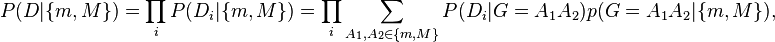$P(D|\{m,M\}) = \prod_i P(D_i|\{m,M\}) =\prod_i \sum_{A_1,A_2 \in \{m,M\}} P(D_i|G=A_1A_2)p(G=A_1A_2|\{m,M\}),$

where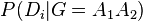$P(D_i|G=A_1A_2)$ is the genotype likelihood. We then assume that the two alleles within an individual are independent and randomly drawn from the set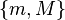$\{m,M\}$ with equal probability, ignoring the fact that the two alleles at a diallelic site are not observed equally frequent. This gives us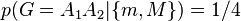$p(G=A_1A_2|\{m,M\})=1/4$ for all four possible combinations of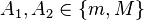$A_1,A_2 \in \{m,M\}$. Therefore we estimate the two possible alleles at the diallelic site by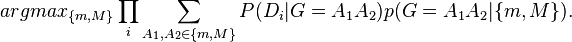$argmax_{\{m,M\}}\prod_i \sum_{A_1,A_2 \in \{m,M\}} P(D_i|G=A_1A_2)p(G=A_1A_2|\{m,M\}).$

To infer which of these two alleles is the minor allele, we estimate the allele frequencies (only one iteration of the EM algorithm is needed if the starting point is frequencies of 0.5).

This is the approach described in Skotte2012.